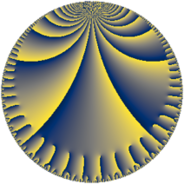# Properties

 Label 112.10.aLevel $112$ Weight $10$ Character orbit 112.a Rep. character $\chi_{112}(1,\cdot)$ Character field $\Q$ Dimension $27$ Newform subspaces $11$ Sturm bound $160$ Trace bound $5$

# Related objects

## Defining parameters

 Level: $$N$$ $$=$$ $$112 = 2^{4} \cdot 7$$ Weight: $$k$$ $$=$$ $$10$$ Character orbit: $$[\chi]$$ $$=$$ 112.a (trivial) Character field: $$\Q$$ Newform subspaces: $$11$$ Sturm bound: $$160$$ Trace bound: $$5$$ Distinguishing $$T_p$$: $$3$$

## Dimensions

The following table gives the dimensions of various subspaces of $$M_{10}(\Gamma_0(112))$$.

Total New Old
Modular forms 150 27 123
Cusp forms 138 27 111
Eisenstein series 12 0 12

The following table gives the dimensions of the cuspidal new subspaces with specified eigenvalues for the Atkin-Lehner operators and the Fricke involution.

$$2$$$$7$$FrickeDim.
$$+$$$$+$$$$+$$$$7$$
$$+$$$$-$$$$-$$$$7$$
$$-$$$$+$$$$-$$$$7$$
$$-$$$$-$$$$+$$$$6$$
Plus space$$+$$$$13$$
Minus space$$-$$$$14$$

## Trace form

 $$27 q + 718 q^{5} - 2401 q^{7} + 204887 q^{9} + O(q^{10})$$ $$27 q + 718 q^{5} - 2401 q^{7} + 204887 q^{9} + 54916 q^{11} + 86158 q^{13} - 81528 q^{15} - 344210 q^{17} + 480888 q^{19} - 812552 q^{23} + 11407757 q^{25} + 5798016 q^{27} + 746738 q^{29} - 6344712 q^{31} + 9585408 q^{33} + 9003750 q^{35} - 2210198 q^{37} - 83320200 q^{39} - 5961738 q^{41} + 83940724 q^{43} + 23553990 q^{45} - 173719272 q^{47} + 155649627 q^{49} + 229586448 q^{51} + 3292082 q^{53} - 246809592 q^{55} + 58487224 q^{57} + 86244480 q^{59} - 90027306 q^{61} - 78764805 q^{63} + 154041124 q^{65} + 181960980 q^{67} - 382360176 q^{69} - 285033976 q^{71} - 471146066 q^{73} - 501618592 q^{75} - 103022108 q^{77} + 978029168 q^{79} + 1432922699 q^{81} + 28717816 q^{83} - 324885860 q^{85} + 4293732080 q^{87} - 388851410 q^{89} + 411449766 q^{91} + 1674528144 q^{93} - 181551768 q^{95} + 344333406 q^{97} + 409926388 q^{99} + O(q^{100})$$

## Decomposition of $$S_{10}^{\mathrm{new}}(\Gamma_0(112))$$ into newform subspaces

Label Dim. $$A$$ Field CM Traces A-L signs $q$-expansion
$$a_2$$ $$a_3$$ $$a_5$$ $$a_7$$ 2 7
112.10.a.a $$1$$ $$57.684$$ $$\Q$$ None $$0$$ $$-170$$ $$544$$ $$2401$$ $$-$$ $$-$$ $$q-170q^{3}+544q^{5}+7^{4}q^{7}+9217q^{9}+\cdots$$
112.10.a.b $$1$$ $$57.684$$ $$\Q$$ None $$0$$ $$6$$ $$560$$ $$2401$$ $$-$$ $$-$$ $$q+6q^{3}+560q^{5}+7^{4}q^{7}-19647q^{9}+\cdots$$
112.10.a.c $$2$$ $$57.684$$ $$\Q(\sqrt{2305})$$ None $$0$$ $$14$$ $$-2730$$ $$-4802$$ $$-$$ $$+$$ $$q+(7+5\beta )q^{3}+(-1365-21\beta )q^{5}+\cdots$$
112.10.a.d $$2$$ $$57.684$$ $$\Q(\sqrt{11209})$$ None $$0$$ $$70$$ $$1554$$ $$4802$$ $$-$$ $$-$$ $$q+(35-\beta )q^{3}+(777+19\beta )q^{5}+7^{4}q^{7}+\cdots$$
112.10.a.e $$2$$ $$57.684$$ $$\Q(\sqrt{193})$$ None $$0$$ $$86$$ $$-2238$$ $$4802$$ $$-$$ $$-$$ $$q+(43+11\beta )q^{3}+(-1119+95\beta )q^{5}+\cdots$$
112.10.a.f $$2$$ $$57.684$$ $$\Q(\sqrt{4561})$$ None $$0$$ $$224$$ $$1596$$ $$-4802$$ $$-$$ $$+$$ $$q+(112-\beta )q^{3}+(798-9\beta )q^{5}-7^{4}q^{7}+\cdots$$
112.10.a.g $$3$$ $$57.684$$ $$\mathbb{Q}[x]/(x^{3} - \cdots)$$ None $$0$$ $$-84$$ $$-2958$$ $$-7203$$ $$+$$ $$+$$ $$q+(-28+\beta _{1})q^{3}+(-986+5\beta _{1}-\beta _{2})q^{5}+\cdots$$
112.10.a.h $$3$$ $$57.684$$ $$\mathbb{Q}[x]/(x^{3} - \cdots)$$ None $$0$$ $$-84$$ $$1554$$ $$-7203$$ $$-$$ $$+$$ $$q+(-28+\beta _{1})q^{3}+(518-6\beta _{1}+7\beta _{2})q^{5}+\cdots$$
112.10.a.i $$3$$ $$57.684$$ $$\mathbb{Q}[x]/(x^{3} - \cdots)$$ None $$0$$ $$92$$ $$274$$ $$7203$$ $$+$$ $$-$$ $$q+(31+\beta _{1})q^{3}+(92+\beta _{1}-\beta _{2})q^{5}+\cdots$$
112.10.a.j $$4$$ $$57.684$$ $$\mathbb{Q}[x]/(x^{4} - \cdots)$$ None $$0$$ $$-84$$ $$1540$$ $$9604$$ $$+$$ $$-$$ $$q+(-21+\beta _{1})q^{3}+(385-2\beta _{1}+\beta _{2}+\cdots)q^{5}+\cdots$$
112.10.a.k $$4$$ $$57.684$$ $$\mathbb{Q}[x]/(x^{4} - \cdots)$$ None $$0$$ $$-70$$ $$1022$$ $$-9604$$ $$+$$ $$+$$ $$q+(-17+\beta _{1})q^{3}+(255-\beta _{1}-\beta _{2}+\cdots)q^{5}+\cdots$$

## Decomposition of $$S_{10}^{\mathrm{old}}(\Gamma_0(112))$$ into lower level spaces

$$S_{10}^{\mathrm{old}}(\Gamma_0(112)) \cong$$ $$S_{10}^{\mathrm{new}}(\Gamma_0(2))$$$$^{\oplus 8}$$$$\oplus$$$$S_{10}^{\mathrm{new}}(\Gamma_0(4))$$$$^{\oplus 6}$$$$\oplus$$$$S_{10}^{\mathrm{new}}(\Gamma_0(7))$$$$^{\oplus 5}$$$$\oplus$$$$S_{10}^{\mathrm{new}}(\Gamma_0(8))$$$$^{\oplus 4}$$$$\oplus$$$$S_{10}^{\mathrm{new}}(\Gamma_0(14))$$$$^{\oplus 4}$$$$\oplus$$$$S_{10}^{\mathrm{new}}(\Gamma_0(16))$$$$^{\oplus 2}$$$$\oplus$$$$S_{10}^{\mathrm{new}}(\Gamma_0(28))$$$$^{\oplus 3}$$$$\oplus$$$$S_{10}^{\mathrm{new}}(\Gamma_0(56))$$$$^{\oplus 2}$$